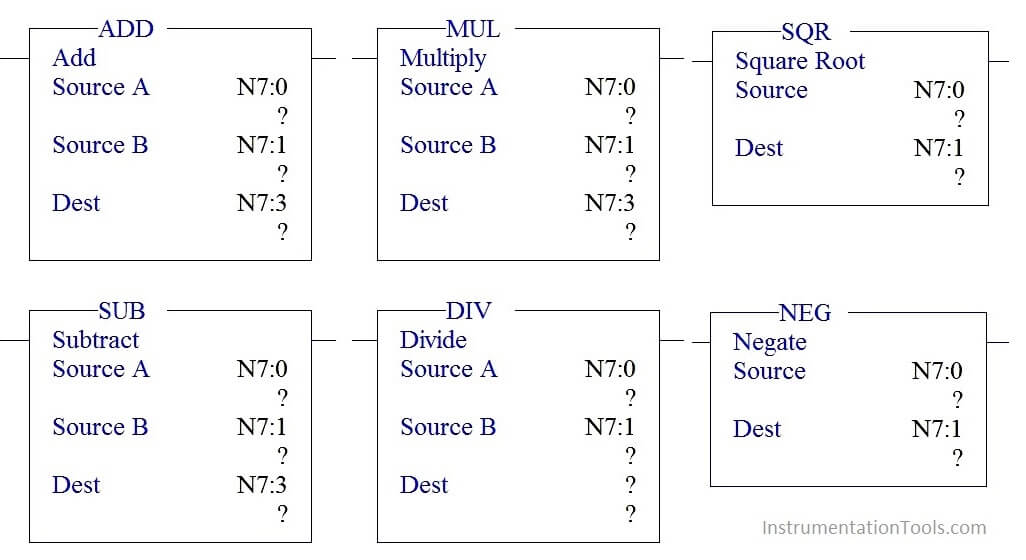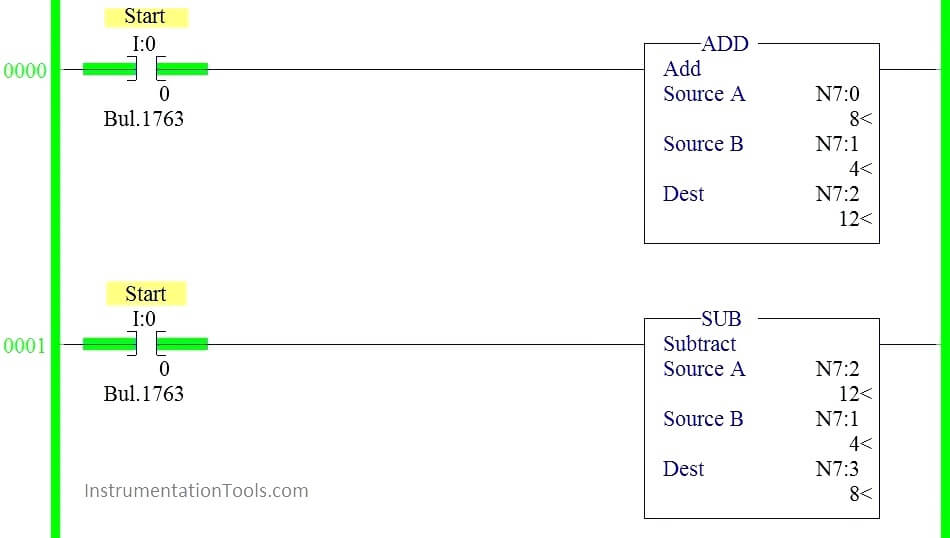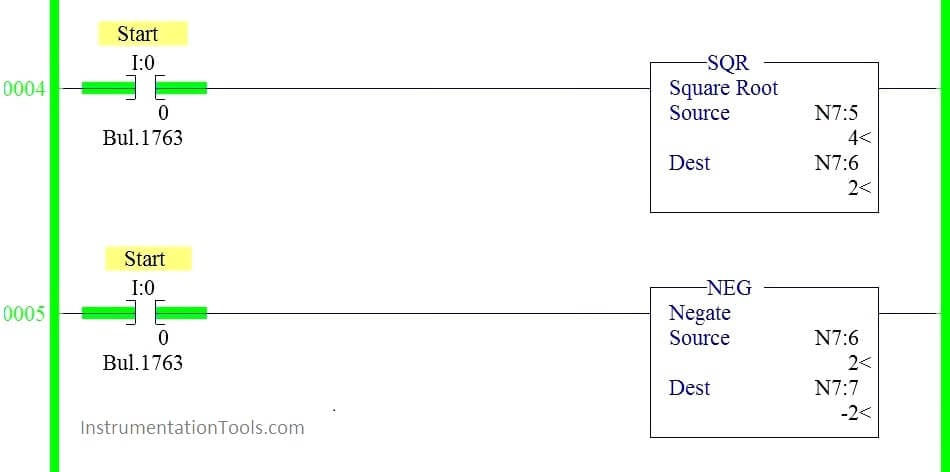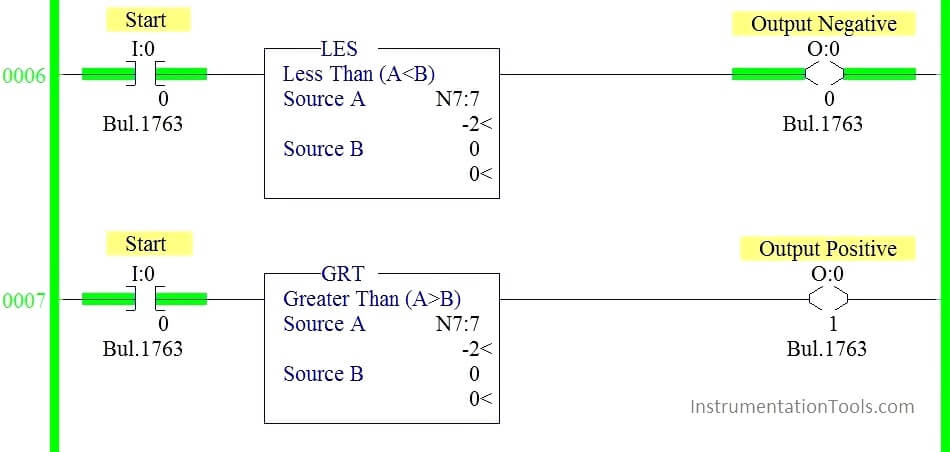Home » Allen Bradley Math Functions
PLC Tutorials

To study the working of Allen Bradley Math functions (ADD, SUB, MUL, DIV, SQR & NEG) using simple example in programmable logical controllers (PLC).There are three parameters in each block,

Source A –Address of the first Value

Source B –Address of the second value

Destination (Dest) – Address where result of the function will store.

## PLC Math Functions

Add block is used to do the Addition of the two values stored in source A and source B and store the output in  destination address.

### SUB:

Sub block is used to do the Subtraction of the two values stored in source A and source B and store the output in destination address.

### MUL:

MUL block is used to do the Multiplication of the two values stored in source A and source B and store the output in destination address.

### DIV:

DIV block is used to do the Division of the two values stored in source A and source B and store the output in destination address.

### SQR

SQR block is used to find the square root of the value stored in source address and results the value in destination address.

### NEQ:

NEQ block is used to negate the value stored in source address and results the value in destination.

## Program Logic

When start switch is pressed,

1. Value stored in N7:0 and N7:1 gets added and results the value in N7:2
2. Add block output is one of the input to Sub block which do subtraction function by taking value stored in N7:1 and stores the address in N7:3.
3. Sub block output is one the input to Mul block and perform multiplication along with value stored in N7:1 and stores the result in N7:4
4. Mul block output is one the input to DIV block and perform Division along with value stored in N7:0 and stores the result in N7:5
5. SQR block performs square root of the Value stored in N7:5 and results the output in N7:6.
6. Value stored in N7:6 get negated using NEG block and results the final output in N7:7.
7. If the resulted output is positive turn on output positive indication else output negative indication.

## PLC Program using Math Functions#### RUNG 0000:

When Start switch is pressed, N7:0 and N7:1 get added and store the value in N7:2.

#### RUNG 0001:

Value stored in N7:2 get subtracted with value stored in N7:1 and results the value in N7:3.

#### RUNG 0002:

Value stored in N7:3 get multiplied with value stored in N7:1 and results the value in N7:4.

#### RUNG 0003:

Value stored in N7:4 get divided by value stored in N7:0 and results the value in N7:5.

#### RUNG 0004:

Value stored in N7:5 get squared and results the value in N7:6.

#### RUNG 0005:

Value stored in N7:6 get negated and results the value in N7:7.

#### RUNG 0006:

Less than block (Source B value sets to 0) is used to check whether the result is negative and results the output in indication lamp (O: 0/0)

#### RUNG 0007:

Greater than block (Source B value sets to 0) is used to check whether the result is positive and results the output in indication lamp (O: 0/1)

### Conclusion:

We can use this example program to understand the working Math block functions in AB PLC.

#### Articles You May Like :

Motor Logic with Push buttons

What is Latching in PLC ?

What is Interlocking in PLC ?

#### PLC Compressor Control Ladder Logic

This website uses cookies to improve your experience. We'll assume you're ok with this, but you can opt-out if you wish. Accept Read More

WordPress Image Lightbox

Send this to a friend